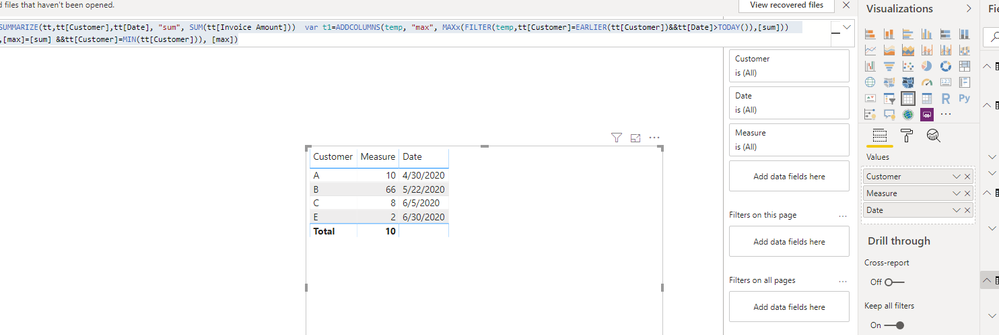cancel
Showing results for
Did you mean:Helper V

## Finding Due Date of Highest Invoice(s) and Amount

Hi all,

Still learining Power BI and not sure where to start with this.  Have a list of customers, due dates and invoice amounts.  I'm looking to have a chart where by it lists all customers, due date of the day where the most is outstanding and also the amount.  I need it not to consider only future values for the next 12 months.  Hope i've explained that okay but added some data below to try and explain better.

 Customer Date Invoice Amount A 17/04/2020 2 B 01/05/2020 3 C 06/05/2020 5 A 17/04/2020 2 A 20/04/2020 3 A 30/04/2020 10 D 15/04/2020 20 **in the past so not considered E 30/06/2020 2 A 17/04/2020 8 B 22/05/2020 66 C 06/05/2020 3 D 19/04/2020 4

 Customer Highest Owed Due Date Amount A 17/04/2020 12 B 22/05/2020 66 C 06/05/2020 8 D 19/04/2020 4 E 30/06/2020 2

Thank you

2 ACCEPTED SOLUTIONSSolution Sage

Hi @NiugeS ,

You can create a calculated column to add the per day total invoice amount for each customer and then use a measure to find the MAX amount of invoice totals. I have created a sample for this which you can download from here.You can use the DAX to create a custom column

``````InvAmount Day = CALCULATE(SUM('Table'[Invoice Amount]),
FILTER('Table', 'Table'[Customer]=EARLIER('Table'[Customer])
&& 'Table'[Date].[Date]=EARLIER('Table'[Date].[Date])))``````

To calculate the measure for Max amount per day use the below DAX as a new measure

``Max Invoice Amount = CALCULATE(MAX('Table'[InvAmount Day]),'Table'[Date]>=TODAY())``

If you need any clarification please do let me know.

If this helped you, please mark this post as an accepted solution and like to give KUDOS .

Regards,

Affan FarooqiCommunity Support

Hi @NiugeS ,

You also could try below measures:

``````Measure =
VAR temp =
SUMMARIZE ( tt, tt[Customer], tt[Date], "sum", SUM ( tt[Invoice Amount] ) )
VAR t1 =
temp,
"max", MAXX (
FILTER ( temp, tt[Customer] = EARLIER ( tt[Customer] ) && tt[Date] > TODAY () ),
[sum]
)
)
RETURN
SUMX (
FILTER ( t1, [max] = [sum] && tt[Customer] = MIN ( tt[Customer] ) ),
[max]
)
``````Best Regards,
Zoe Zhi

If this post helps, then please consider Accept it as the solution to help the other members find it more quickly.

3 REPLIES 3Community Support

Hi @NiugeS ,

You also could try below measures:

``````Measure =
VAR temp =
SUMMARIZE ( tt, tt[Customer], tt[Date], "sum", SUM ( tt[Invoice Amount] ) )
VAR t1 =
temp,
"max", MAXX (
FILTER ( temp, tt[Customer] = EARLIER ( tt[Customer] ) && tt[Date] > TODAY () ),
[sum]
)
)
RETURN
SUMX (
FILTER ( t1, [max] = [sum] && tt[Customer] = MIN ( tt[Customer] ) ),
[max]
)
``````Best Regards,
Zoe Zhi

If this post helps, then please consider Accept it as the solution to help the other members find it more quickly.Solution Sage

Hi @NiugeS ,

You can create a calculated column to add the per day total invoice amount for each customer and then use a measure to find the MAX amount of invoice totals. I have created a sample for this which you can download from here.You can use the DAX to create a custom column

``````InvAmount Day = CALCULATE(SUM('Table'[Invoice Amount]),
FILTER('Table', 'Table'[Customer]=EARLIER('Table'[Customer])
&& 'Table'[Date].[Date]=EARLIER('Table'[Date].[Date])))``````

To calculate the measure for Max amount per day use the below DAX as a new measure

``Max Invoice Amount = CALCULATE(MAX('Table'[InvAmount Day]),'Table'[Date]>=TODAY())``

If you need any clarification please do let me know.

If this helped you, please mark this post as an accepted solution and like to give KUDOS .

Regards,

Affan FarooqiSuper User

Become an expert!: Enterprise DNA
External Tools: MSHGQM
Latest book!:
Mastering Power BI 2nd EditionDAX is easy, CALCULATE makes DAX hard...